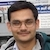# NAEST 2019 Screening Test: Pull an Inverted Mug from the WaterBy

Immerse a mug in the bucket of water and invert it i.e., make the mug upside down. Now, hold the mug from its bottom side and starts pulling it up. The force applied by the hand is very small when the mug is completely immersed in the water. This force increases as the mug start coming out of the water. Let us explain this observation.

Question: The mass of the empty plastic mug is a few grams. The boy is struggling hard to pull the inverted mug from the bucket of water. Assume that the boy pulls up the mug very slowly. Choose the correct option(s)

1. The adhesive forces between mug and water are responsible for the high pulling force by the boy.
2. The pulling force by the boy increases linearly with height of the mug above the water surface.
3. The atmospheric air pushes the mug downwards and the air (trapped) in the mug pushes the mug upwards. The difference between these forces is approximately equal to the pulling force by the boy.
4. The boy is lying, it is very easy to pull the mug.

Solution: Let $\rho$ be the density of the water and $h_0$ be the total height of the mug of the cross-sectional area $A$. The mug is completely filled with water because it is inverted after immersing it in the water. However, there is a small amount of air trapped inside the mug near its base. The volume of this air is very small. Let height $h$ of the mug is outside the water.Let $p_0$ be atmospheric pressure and $p$ be the pressure of the trapped air (when the height $h$ of the mug is outside the water). The forces acting on the mug are

1. The force applied by the hand ($F$) in the upward direction
2. The gravitational pull ($mg$) in the downward direction
3. The force due to atmospheric air ($p_0A$) in the downward direction
4. The force due to trapped air ($pA$) in the upward direction

The force in the horizontal direction (due to water and air) cancels out due to symmetry. The acceleration of the mug is zero because it is pulled out slowly at a constant speed (assumed). Thus, the net force on the mug is zero i.e., \begin{align} F=(p_0-p)A+mg \end{align}

The pressure of trapped air $p$ is equal to the difference of atmospheric pressure and hydrostatic pressure due to water column above the water surface i.e., \begin{align} p=p_0-\rho g h \end{align}

Eliminate $p$ from the above equations to get \begin{align} F=\rho g h + mg \end{align} Thus, applied force increases linearly with the height of the mug above the water surface. This force attains a maximum value $\rho g h_0+mg$ i.e., the weight of the mug completely filled with water. After that, the air enters into the mug and the water spills out.